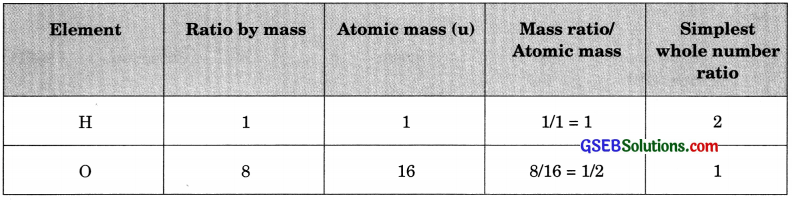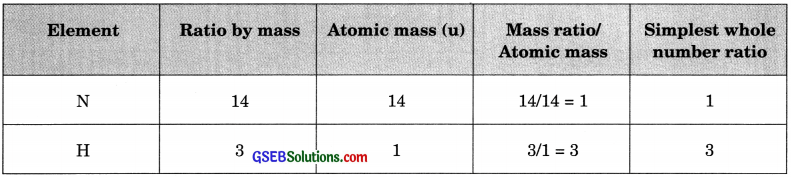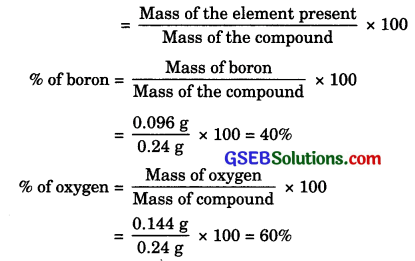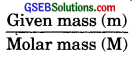# GSEB Solutions Class 9 Science Chapter 3 Atoms and Molecules

Gujarat Board GSEB Textbook Solutions Class 9 Science Chapter 3 Atoms and Molecules Textbook Questions and Answers, Notes Pdf.

## Gujarat Board Textbook Solutions Class 9 Science Chapter 3 Atoms and Molecules

### Gujarat Board Class 9 Science Atoms and Molecules InText Questions and Answers

Page – 32

Question 1.
In a reaction, 5.3 g of sodium carbonate were reacted with 6 g of ethanoic acid solution. The products were 2.2 g of carbon dioxide, 0.9 g of water and 12 g of sodium ethanoate solution. Show that these observations are in agreement with the law of conservation of mass.
Sodium carbonate + Ethanoic acid → Sodium ethanoate + Carbon dioxide + Water
Mass of reactants = Mass of sodium carbonate + Mass of ethanoic acid
= 5.3 + 6.0 = 11.3 g
Mass of products = Mass of carbon dioxide + Mass of water + Mass of sodium ethanoate
= 2.2 + 0.9 + 8.2 = 11.3 g
Since the mass of products is equal to mass of reactants, therefore mass is neither created nor lost during the given chemical change or the observation made is in agreement with the law of conservation of mass.Question 2.
Hydrogen and oxygen combine in the ratio 1 : 8 by mass to form water. What mass of oxygen gas would be required to react completely with 3 g of hydrogen gas?
As hydrogen and oxygen combine in the ratio of 1 : 8 by mass, this means that 1 g of hydrogen combines with 8 g of oxygen.
∴ 3 g of hydrogen will react with oxygen = 8 x 3 g = 24gQuestion 3.
Which postulate of Dalton’s atomic theory is the result of the law of conservation of mass?
The postulate of Dalton’s Atomic theory that explain the law of conservation of mass is ‘Atoms are indivisible particles, which cannot be created or destroyed in a chemical reaction’.

Question 4.
Which postulate of Dalton’s atomic theory can explain the law of definite proportions?
The following postulate of Dalton’s atomic theory can explain the law of definite proportions: The relative number and kinds of atoms are constant in a given compound.

Page – 35

Question 5.
Define the atomic mass unit.
Mass unit equal to exactly one-twelfth the mass of one atom of carbon-12 is called one atomic mass unit.

Question 6.
Why is it not possible to see an atom with naked eyes?
The size of an atom is very small. Further atoms of most elements do not exist independently. The radius of an atom is of the order of 10-10 m.Page – 39

Question 7.
Write down the formulae of
(i) sodium oxide
(ii) aluminium chloride
(iii) sodium sulphide
(iv) magnesium hydroxide
(i) Na2O
(ii) A2C3
(iii) Na2S
(iv) Mg(OH)2

Question 8.
Write down the names of compounds represented by the following formulae:
(i) Al2(SO4)3
(ii) CaCl2
(iii) K2SO4
(iv) KNO3
(v) CaCO3
(i) Aluminium sulphate
(ii) Calcium chloride
(iii) Potassium sulphate
(iv) Potassium nitrate
(v) Calcium carbonate

Question 9.
What is meant by the term chemical formula?
Chemical formula of a compound means the symbolic representation of the composition of a compound. From the chemical formula of a compound, we can know the number and kinds of atoms of different elements that constitute the compound. For example, from the chemical formula of carbon dioxide (CO2), we come to know that one carbon atom and two oxygen atoms are chemically bonded together to form one molecule of the compound, carbon dioxide.

Question 10.
How many atoms are present in a
(i) H2S molecule and
(ii) PO43- ion?
(i) In H2S molecule, there are three atoms.

(ii) In PO43- ion, there are five atoms.Page – 40

Question 11.
Calculate the molecular masses of H2, O2, Cl2, CO2, CH4, C2H6, C2H4, NH3, CH3OH.
Molecular mass of H2 = 2 x Atomic mass of H
= 2 x 1 = 2 u
Molecular mass of O2 = 2 x Atomic mass of O
= 2 x 16 = 32 u
Molecular mass of Cl2 = 2 x Atomic mass of Cl
= 2 x 35.5 = 71 u
Molecular mass of CO2 = Atomic mass of C + 2 x Atomic mass of O
= 12 + 2 x 16 = 44 u
Molecular mass of CH4 = Atomic mass of C + 4 x Atomic mass of H
= 12 + 4 x 1 = 16 u
Molecular mass of C2H6 = 2 x Atomic mass of C + 6 x Atomic mass of H
= 2 x 12 + 6 x 1 = 30u
Molecular mass of C2H4 = 2 x Atomic mass of C + 4 x Atomic mass of H
= 2 x 12 + 4 x 1 = 28u
Molecular mass of NH3 = Atomic mass of N + 3 x Atomic mass of H
= 14 + 3 x 1 = 17 u
Molecular mass of CH3OH = Atomic mass of C + 4 x Atomic mass of H + Atomic mass of O.
= 12 + 4 x 1 + 16 = 32 u

Question 12.
Calculate the formula unit masses of ZnO, Na2O, K3CO3 given atomic masses of Zn = 65 u, Na = 23 u, K = 39 u, C = 12 u and O = 16 u.
Formula unit mass of ZnO = 65 + 16 = 81 u
Formula unit mass of Na2O = 23 x 2 + 16 = 62
u Formula unit mass of K2CO3 = 39 x 2 + 12 x 1 + 16 x 3 = 138 u

Page – 42

Question 13.
If one mole of carbon atoms weighs 12 gram, what is the mass (in grams) of 1 atom of carbon?
One mole of carbon atom weighs 12 g (Given) i.e., mass of 1 mole of carbon atoms = 12 g
Then, mass of 6.022 x 1023 number of carbon atoms = 12 g
Therefore, mass of 1 atom of carbon =$$\frac{6.022×10^{23}×100}{23}$$ = 1.9926 x 10-23g

Question 14.
Which has more number of atoms, 100 grams of sodium or 100 grams of iron (given, atomic mass of Na = 23 u, Fe = 56 u)?
Atomic mass of Na = 23 u (Given)
Then, gram atomic mass of Na = 23 g
Now, 23 g of Na contains = 6.022 x 1023 number of atoms
Thus, 100 g of Na contains = $$\frac{6.022×10^{23}×100}{23}$$
= 2.6182 x 1024 number of atoms
Again atomic mass of Fe = 56 u
Then, gram atomic mass of Fe = 56 g
Now, 56 g of Fe contains = 6.022 x 1023 number of atoms
Thus, 100 g of Fe contains = $$\frac{6.022×10^{23}×100}{56}$$ number of atoms
= 1.0753 x 1024 number of atoms
Therefore, 100 grams of sodium contain more number of atoms than 100 grams of iron.In-Text Activities Solved

(Textbook Page 1)

Activity 3.1
Observation: On tilting the flask the solution X in an ignition tube and Y solution gets mixed. Yes the chemical reaction has taken place. A cork was put on the mouth of the conical flask to ensure there is no loss in the mass by completion of chemical reaction. No, there is no change in the mass of the flask according to law of mass conservation.

Conclusion: Law of conservation of mass states that “mass can neither be created nor destroyed during the course of chemical reaction”.

(Textbook Page 36)

Activity 3.2
Calculations on the basis of observations of Table 3.4.
(a) The ratio by number of atoms for a water molecule can be found as follows:(b) The ratio by number of atoms for a ammonia molecule can be found as follows:(c) The ratio by number of atoms for a carbon dioxide molecule can be found as follows:Conclusion:
(a) The ratio by number of atoms for H : O = 2 : 1 = H2O
(b) The ratio by number of atoms for N : H = 1 : 3 = NH3
(c) The ratio by number of atoms for C : O = 1 : 2 = CO2

### Gujarat Board Class 9 Science Atoms and Molecules Textbook Questions and Answers

Question 1.
A 0.24 g sample of compound of oxygen and boron was found by analysis to contain 0.096 g of boron and 0.144 g of oxygen. Calculate the percentage composition of the compound by weight.
% of any element in a compound –Question 2.
When 3.0 g of carbon is burnt in 8.00 g oxygen, 11.00 g of carbon dioxide is produced. What mass of carbon dioxide will be formed when 3.00 g of carbon is burnt in 50.00 g of oxygen?
When 3.0 g carbon is burnt in 8.00 g oxygen, 11.00 g carbon dioxide is formed. It means all of the carbon and oxygen are used up and carbon and oxygen are combined in the ratio of 3 : 8 to form carbon dioxide. Thus when there is 3 g carbon and 50.0 g oxygen, then also only 8 g oxygen will be used and 11.0 g carbon dioxide will be formed. The remaining oxygen is not used up. This indicates law of definite proportions which says that in compounds, the combining elements are present in definite proportions by mass.

Question 3.
What are polyatomic ions? Give examples.
A polyatomic ion is a group of atoms carrying a charge (positive or negative). For example, ammonium ion (NH4+), hydroxide ion (OH), carbonate ion (CO32-), sulphate ion (SO42-).

Question 4.
Write the chemical formulae of the following.
(a) Magnesium chloride
(b) Calcium oxide
(c) Copper nitrate
(d) Aluminium chloride
(e) Calcium carbonate
(a) MgCl2
(b) CaO
(c) Cu(NO3)2
(d) AlCl3
(e) CaCO3Question 5.
Give the names of the elements present in the following compounds.
(a) Quicklime
(b) Hydrogen bromide
(c) Baking powder
(d) Potassium sulphate
(a) Calcium and oxygen
(b) Hydrogen and bromine
(c) Sodium, Hydrogen, Carbon and Oxygen
(d) Potassium, Sulphur and Oxygen.

Question 6.
Calculate the molar mass of the following substances.
(a) Ethyne, C2H2
(b) Sulphur molecule, S8
(c) Phosphorus molecule, P4 (Atomic mass of phosphorus = 31)
(d) Hydrochloric acid, HCl
(e) Nitric acid, HNO3
(a) Ethyne, C2H2 = 2 x 12 + 2 x 1 = 26g
(b) Sulphur molecule, S8 = 8 x 32 = 256 g
(c) Phosphorus molecule, P4 = 4 x 31 = 124 g
(d) Hydrochloric acid, HCl = 1 x 1 + 1 x 35.5 = 36.5 g
(e) Nitric acid, HNO3 = 1 x 1 + 1 x 14 + 3 x 16 = 63g

Question 7.
What is the mass of:
(а) 1 mole of nitrogen atom?
(b) 4 moles of aluminium atoms (Atomic mass of aluminium = 27)?
(c) 10 moles of sodium sulphite (Na2SO3)?
(a) The mass of 1 mole of nitrogen atoms is 14 g.

(b) The mass of 4 moles of aluminium atoms is (4 x 27) g = 108 g

(c) The mass of 10 moles of sodium sulphite (Na2SO3) is 10 x [2 x 23 + 32 + 3 x 16] g
= 10 x 126 g = 1260 g.

Question 8.
Convert into mole.
(a) 12 g of oxygen gas
(b) 20 g of water
(c) 22 g of carbon dioxide
(a) No. of moles (n) =Molar mass of O2 = 2 x 16 g mol-1 = 32 g mol-1
∴ No.of moles in 12 g O2 = $$\frac { 12g }{ { 32gmol }^{ -1 } }$$ = 0.375 mole.

(b) Molar mass of H2O = 2 x 1.0 + 16 g mol-1 = 18 g mol-1
∴ No.of moles in 20 g H2O = $$\frac { 20g }{ { 18gmol }^{ -1 } }$$ = 1.11 mole.

(c) Molar mass of CO2 = 12 + 2 x 16 g mol-1 = 44 g mol-1
∴ No.of moles in 22 g CO2 = $$\frac { 22g }{ { 44gmol }^{ -1 } }$$ = 0.5 mole.

Question 9.
What is the mass of:
(a) 0.2 mole of oxygen atoms?
(b) 0.5 mole of water molecules?
(a) Mass of one mole of oxygen atoms = 16 g
Then, mass of 0.2 mole of oxygen atoms = 0.2 x 16 g = 3.2 g.

(b) Mass of one mole of water molecule = 18 g
Then, mass of 0.5 mole of water molecules = 0.5 x 18 g = 9 g.Question 10.
Calculate the number of molecules of sulphur (S8) present in 16 g of solid sulphur.
1 mole of S8 = 32 x 8 = 256 g
1 mole of S8 = 6.023 x 1023 molecules
256 g S8 has = 6.023 x 1023 S8 molecules
16 g S8 has = $$\frac{6.023×{10}^{23}}{256}$$
= 3.76 x 1022 molecules

Question 11.
Calculate the number of aluminium ions present in 0.051 g of aluminium oxide.
(Hint: The mass of an ion is the same as that of an atom of the same element. Atomic mass of Al = 27u)
0.051 g Al2O3 has = $$\frac { 6.023\times { 10 }^{ 23 }\times 0.051 }{ 102 }$$